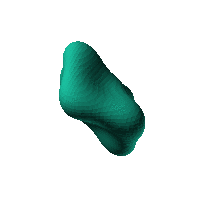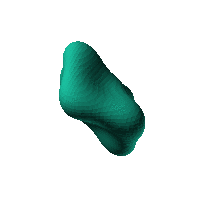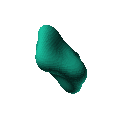Blob Farm# blob21The blob in all its glory:4 Blob with 3 fold symmetry.

$0.005\text{}{\left({x}^{2}+{y}^{2}+{z}^{2}\right)}^{3}+{x}^{3}y+x{z}^{3}+{y}^{3}z<70$

 Click on the snapshot to download the blob's stl file.Octave Code:
```1; # Prevent Octave from thinking that this is a function
# though one is defined here

function w = f(x2,y2,z2,c,r,e)
x  = (x2-c(1))/r(1);
y  = (y2-c(2))/r(2);
z  = (z2-c(3))/r(3);
# function at origin must be <0, and >0 far enough away.  w=0 defines the surface
w=(x.^3.*y + y.^3.*z + z.^3.*x ) + 0.005*(x.^2 + y.^2 + z.^2).^3 - 70 ;

endfunction;

```
GNU Octave